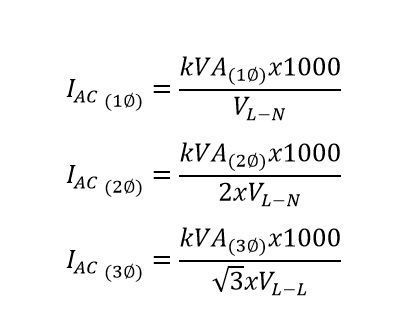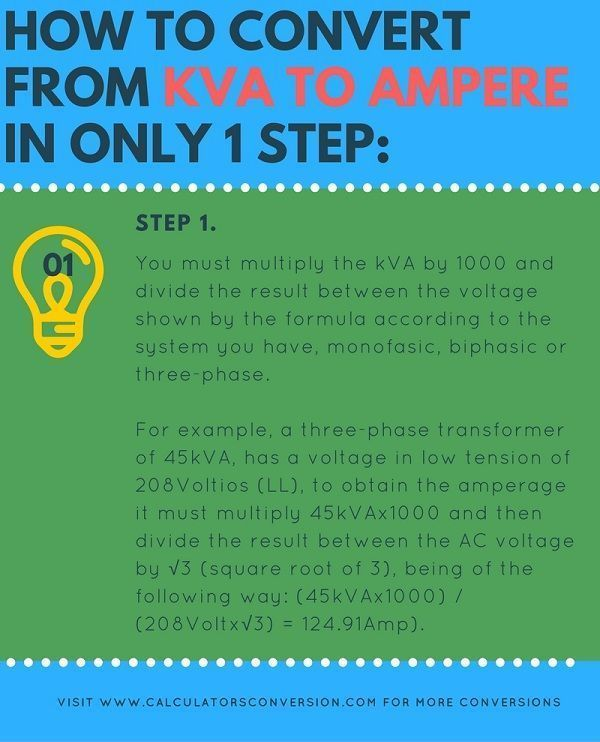# kVA to Amps – Calculators, formula, chart, convert and transformer free.

With this calculator you can convert from kVA to Amps easily, quickly and free any electric power.

For greater ease we explain that formula is used for the calculation, how to perform the conversion in only 1 step , some examples and a table with the main conversions from kVA to Amperes.

## kVA to Amps calculation formula for generator and transformer:• AC = Amps.
• LL = Line-Line Volts.
• LN = Line-Neutral Volts.
• AC1Ø = Current / Amps 1 phase.
• AC2Ø = Current / Amps 2 phases.
• AC3Ø = Current / Amps 3 phases.
• (kVA) = Kilovoltio-Amperes.

## How to convert from kVA to Ampere in only 1 step:Step 1:

You must multiply the kVA by 1000 and divide the result between the voltage shown by the formula according to the system you have, monofasic, biphasic or three-phase.

For example, a three-phase transformer of 45kVA, has a voltage in low tension of 208Voltios (LL), to obtain the amperage it must multiply 45kVAx1000 and then divide the result between the AC voltage by √3 (square root of 3), being of the following way: (45kVAx1000) / (208Voltx√3) = 124.91Amp).

## Examples of kVA to Amps conversions:

Example 1:

A mill of 120 Volts (LN) has a power of 4kVA,  how many amps does it have?.

Rta: // First you must choose the indicated formula, which will be for monophasic equipment that is: kVAx1000 / V LN then simply replace the values ​​in the formula, leaving as follows: 4kVAx1000 / 120Voltios, the result will be: 33.33Ampere

Example 2:

A three-phase food crusher has a power of 8kVA and a voltage of 220Volts, how many amps does the crusher have?

Rta: // You must multiply initially 8kVAx1000, and the result must be divided by 220Vx√3, resulting in: 20,99Amp.

Example 3:

A biphasic industrial blender, has a power of 2,5kVA and a line-to-line voltage of 240Volts and a line-neutral voltage of 120Volts, what amperage does the blender have ?.

Rta: // Simply enter the information supplied to the calculator, power: 2,5kVA, Line-Neutral voltage: 120Volts, biphasic (2F), the result will be: 10.42Ampere.

## kVA to amperes, table for conversion, equivalence, transformation (3F, 220Volt) :

 How many kVA are: Ampere Equivalence 1 kVA Equivalent to 2.62 Amp. 2 kVA 5.25 Amp. 3 kVA 7.87 Amp. 4 kVA 10.50 Amp. 5 kVA 13.12 Amp. 6 kVA 15.75 Amp. 7 kVA 18.37 Amp. 8 kVA 20,99 Amp. 9 kVA 23.62 Amp. 10 kVA 26.24 Amp. 20 kVA 52.49 Amp. 30 kVA 78.73 Amp. 40 kVA 104.97 Amp. 50 kVA 131.22 Amp. 60 kVA 157.46 Amp. 70 kVA 183.70 Amp. 80 kVA 209.95 Amp. 90 kVA 236.19 Amp. 100 kVA 262.43 Amp. 200 kVA 524.86 Amp. 300 kVA 787.30 Amp. 400 kVA 1049.73 Amp. 500 kVA 1312.16 Amp. 600 kVA 1574.59 Amp. 700 kVA 1837.02 Amp. 800 kVA 2099.46 Amp. 900 kVA 2361.89 Amp. 1000 kVA 2624.32 Amp. 2000 kVA 5248.64 Amp. 3000 kVA 7872.96 Amp. 4000 kVA 10497.28 Amp. 5000 kVA 13121.60 Amp. 6000 kVA 15745.92 Amp. 7000 kVA 18370.24 Amp.

Note: the previous table of conversions only applies for three-phase loads (3F) with a voltage of 220Volts, for variables different from the previous ones, the calculator should be used.

## How to use the calculator from kVA to Amps:

Initially you must enter the kVA you want to convert to amps, then you must choose the number of phases 1,2,3 (Monofasic, biphasic or three phase) (It is important that once you choose the number of phases, be aware of the voltage to enter, LL or LN, this depends on the correct information delivered by the tool) , then you must enter the voltage of the equipment and finally click on calculate, if you want to enter a new information you just have to click on restart.

Rate this calculator kVA to Amps:  [kkstarratings]